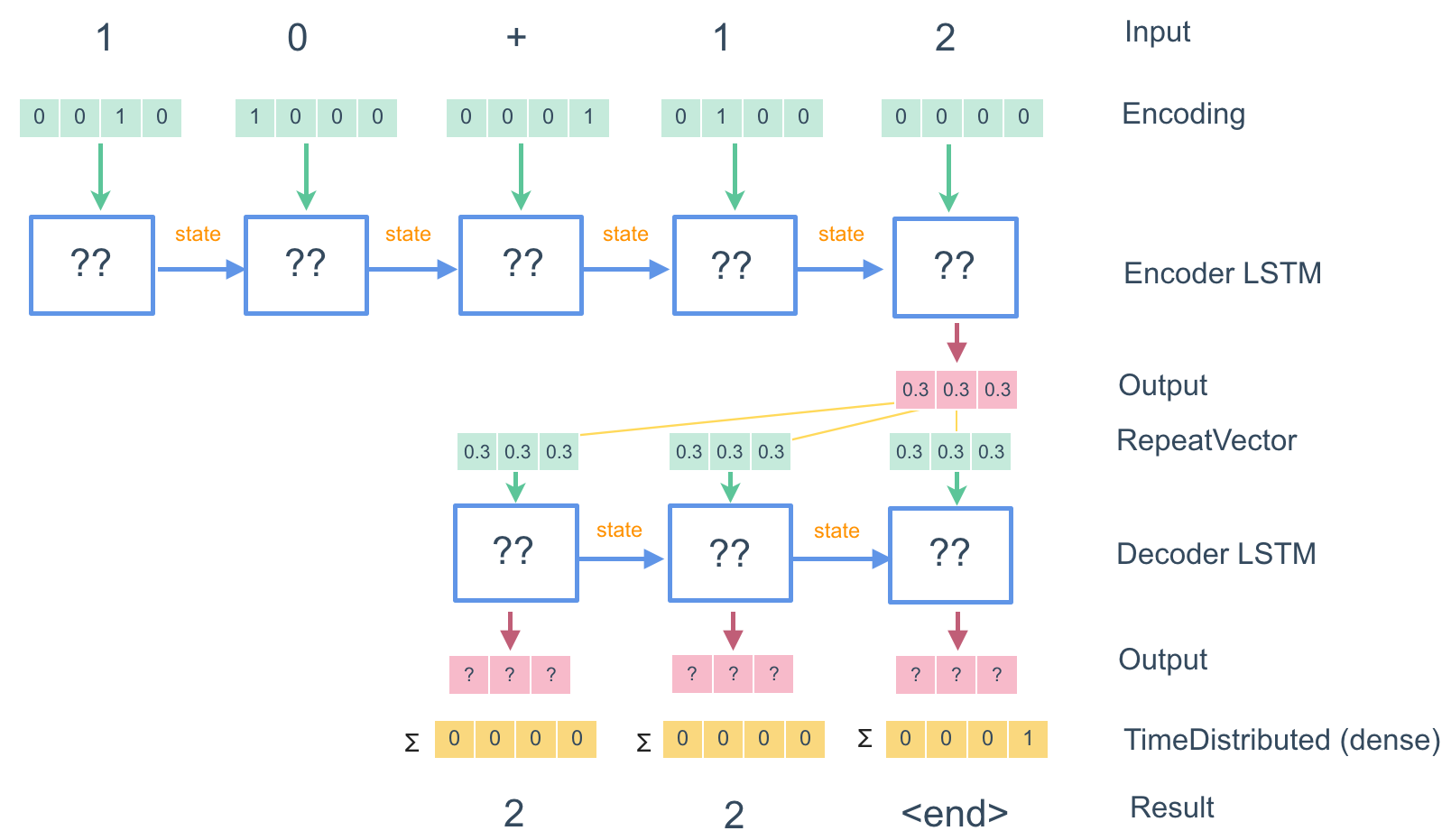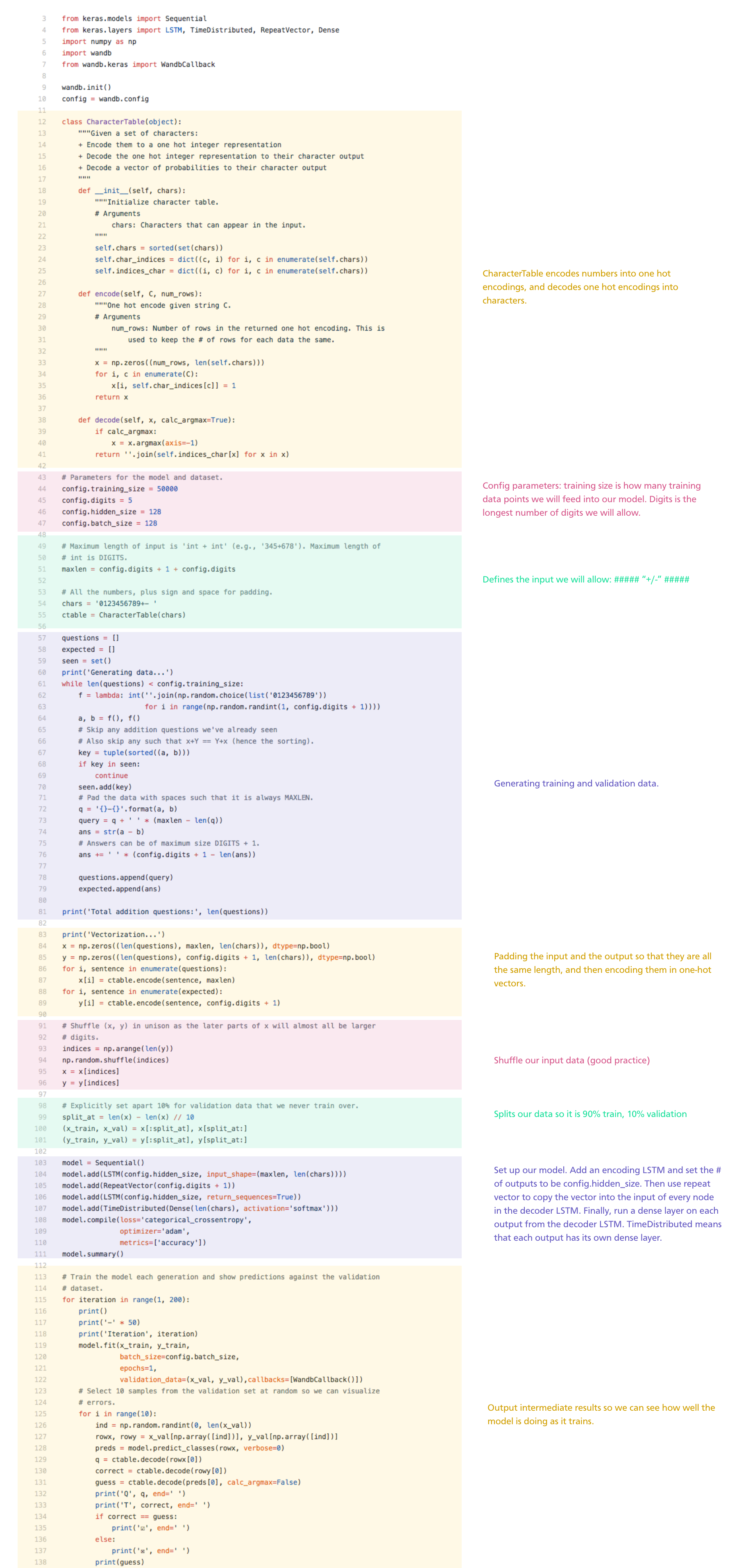# 10. Seq2Seq

In this tutorial, we are going to look at one of the coolest applications of LSTMs: Seq2Seq models. The canonical example of Seq2Seq is translation, and in fact Seq2Seq models are what Google Translate uses.

We are going to build a Seq2Seq model that takes in strings of arithmetic equations (e.g. “10 + 12”) and returns the answer to that equation (“22”). What makes this really amazing is that the model knows nothing about arithmetic - it is ‘translating’ the strings.

# How does Seq2Seq work

Let’s go through how the LSTM works on our simple “10 + 12” = “22” model.• Firstly, we take the digits (and arithmetic operators e.g. +) and character encode them into a one-hot encoding.
• Next, we feed that list of arrays into an “encoder” LSTM. This LSTM tries to learn some sort of encoding of the input.
• We then take the final output of the LSTM, and use it as input into all of the nodes on a “decoder” LSTM (we will use Keras’ RepeatVector function to do this).
• At each node, the decoder LSTM takes in the output of the encoder LSTM as input, as well as the state passed to it by the previous node in the decoder LSTM.
• We then take the final output of the LSTM, and use it as input into all of the nodes on a “decoder” LSTM (we will use Keras’ RepeatVector function to do this).The decoder LSTM outputs an arbitrary length string, and when it is done, it outputs a special <end> character</end>
• When we see the <end> character, we take the output at each step from the decoder LSTM, run it through a dense layer, and translate the one-hot encoded vectors back into characters.</end>

# Code

Go into the seq2deq directory and open up train.py. Here you will see the full code for implementing our arithmetic model.Congratulations! You have finished this course, and learned a ton about the inner workings of RNNs, LSTMs, word embeddings and Seq2Seq. You’re now ready to try building your own models based on code shown in these classes.

Congratuations! You have finished this course.